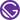# Building a Stack Class in Java

February 06, 2020

A stack is a fundamental data structure in programming. It behaves like a data container where new items are added to the top of the stack and you only have access to last one added (most top item).

A definition by Oracle

The Stack class represents a last-in-first-out (LIFO) stack of objects. The usual push and pop operations are provided, as well as a method to peek at the top item on the stack, a method to test for whether the stack is empty, and a method to search the stack for an item and discover how far it is from the top.

Today, we try to recreate this data structure in Java with our own generic class and interface. The class is generic in order to store different data types. It should provide the following six methods:

• push (to add a new item to the top)
• pop (to remove the most top item)
• peek (to get the most top item)
• isEmpty (to check whether the stack is empty)
• size (to get the size of the stack)
• search (to search for objects)

### Interface

Let’s start with the interface for our stack. The interface specifies which methods have to be implemented inside the stack class. We declare the six methods I just mentioned before.

The `<T>` construct marks the interface as generic. In this case `T` can be any data type. For example `Integer` or `String`.

``````interface Stackable<T> {
void push(T value);
T pop();
T peek();
boolean isEmpty();
int size();
int search(Object o);
}``````

### Class

As next, we create the stack class. Here we need two private attributes: `previous` and `value`.

`previous` is a reference to the item that is located below the current instance in the stack order (the underlying item). This results in a recursive implementation.

The `value` attribute contains the value that the current instance of stack stores. It can be any data type.

Moreover, there are multiple constructors we need later on. Since the class is generic we need here the `<T>` construct as well.

``````class Stack<T> implements Stackable<T> {
private Stack<T> previous;
private T value;

Stack() {
}

Stack(T value) {
this.value = value;
}

Stack(Stack<T> previous, T value) {
this.previous = previous;
this.value = value;
}
}``````

#### Method: push

This method pushes a new item onto the top of the stack. Therefore, we set the current instance of stack to our previous one and store the new value.

`previous` references now to our old stack instance.

``````@Override
public void push(T value) {
if (this.value == null)
this.value = value;
else {
this.previous = new Stack<T>(this.previous, this.value);
this.value = value;
}
}``````

#### Method: pop

This method removes the item at the stack’s top and returns its value.

First of all we store the current value in a temporary variable because it gets overwritten and we need to return it later.

Afterwards, we set the current `value` to the one from our `previous` stack item. Moreover, we reference the current `previous` attribute to the `previous` item of the underlying item.

At the end, we return the removed value.

``````@Override
public T pop() {
if (this.isEmpty())
throw new IllegalArgumentException("Stack is empty");

T top = this.value;
this.value = this.previous.value;
this.previous = this.previous.previous;

}``````

#### Method: peek

This method looks at the value of the item at the top of the stack and returns it. Here we just need to return `value`.

``````@Override
public T peek() {
return this.value;
}``````

#### Method: isEmpty

This method tests if the stack is empty. Since the last stack item has no reference to another (underlying) item, we just need to check if the `previous` item is `null`.

``````@Override
public boolean isEmpty() {
return this.previous == null;
}``````

#### Method: size

This method returns number of items in our stack. Here, we recursively count until the last item is reached.

``````@Override
public int size() {
return this.isEmpty() ? 0 : 1 + this.previous.size();
}``````

#### Method: search

This method returns the 1-based position where an item is on the stack. Therefor, we loop over all our stack items and increase a counter until the one that equals the target item is reached. Last but not least we return the counter.

If there is no match, `-1` is returned.

``````@Override
public int search(Object o) {
int count = 1;

for (Stack<T> stack = this; !stack.isEmpty(); stack = stack.previous) {
if (stack.value.equals(o))
return count;
count++;
}

return -1;
}``````

#### Method: toString

This method is used to represent the Stack as String.

``````@Override
public String toString() {
if (!this.isEmpty())
return this.previous + " <- " + this.value;
return this.value != null ? String.valueOf(this.value) : "";
}``````

## Try it out

Let’s create a new instance of our `Stack` class and try it out.

``````Stack<Integer> stack = new Stack<Integer>();

System.out.println(stack.isEmpty());    // true

stack.push(2);
stack.push(5);
stack.push(7);
stack.push(16);

System.out.println(stack.isEmpty());    // false

System.out.println(stack.size());       // 4

System.out.println(stack.peek());       // 16

System.out.printl(stack.pop());         // 16

System.out.println(stack.peek());       // 7

System.out.println(stack.search(16));   // -1
System.out.println(stack.search(5));    // 2
System.out.println(stack.search(7));    // 1``````

That’s it! Here you can find a GitHub Gist including the complete code.This is my personal blog where I mostly write about technical or computer science based topics. Check out my GitHub profile too.